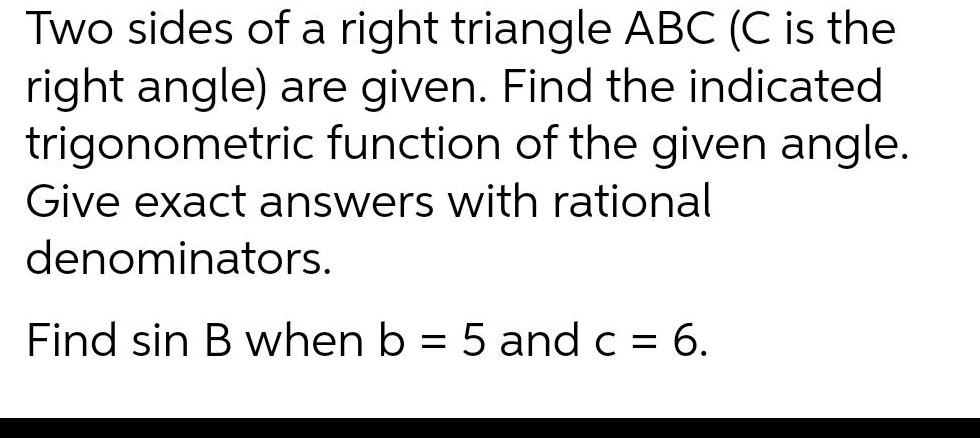Question:

# Two sides of a right triangle ABC (C is the right angle) are

Last updated: 7/6/2022Two sides of a right triangle ABC (C is the right angle) are given. Find the indicated trigonometric function of the given angle. Give exact answers with rational denominators. Find sin B when b = 5 and c = = 6.